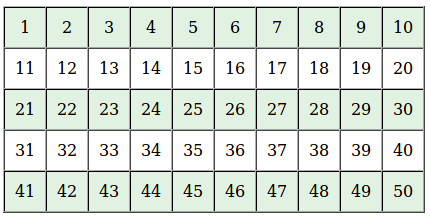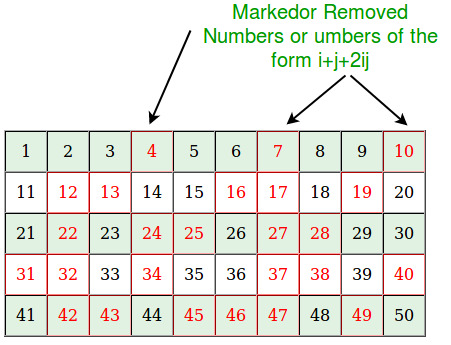# Sieve of Sundaram to print all primes smaller than n

Given a number n, print all primes smaller than or equal to n.

Examples:

```Input:  n = 10
Output: 2, 3, 5, 7

Input:  n = 20
Output: 2, 3, 5, 7, 11, 13, 17, 19```

We have discussed Sieve of Eratosthenes algorithm for the above task.

Below is Sieve of Sundaram algorithm.

```printPrimes(n)
[Prints all prime numbers smaller than n]

1) In general Sieve of Sundaram, produces primes smaller than
(2*x + 2) for given number x. Since we want primes
smaller than n, we reduce n-1 to half. We call it nNew.
nNew = (n-1)/2;
For example, if n = 102, then nNew = 50.
if n = 103, then nNew = 51

2) Create an array marked[n] that is going
to be used to separate numbers of the form i+j+2ij from
others where  1 <= i <= j

3) Initialize all entries of marked[] as false.

4) // Mark all numbers of the form i + j + 2ij as true
// where 1 <= i <= j
Loop for i=1 to nNew
a) j = i;
b) Loop While (i + j + 2*i*j)  2, then print 2 as first prime.

6) Remaining primes are of the form 2i + 1 where i is
index of NOT marked numbers. So print 2i + 1 for all i
such that marked[i] is false. ```

Below is the implementation of the above algorithm :

## C++

 `// C++ program to print primes smaller than n using` `// Sieve of Sundaram.` `#include ` `using` `namespace` `std;`   `// Prints all prime numbers smaller` `int` `SieveOfSundaram(``int` `n)` `{` `    ``// In general Sieve of Sundaram, produces primes smaller` `    ``// than (2*x + 2) for a number given number x.` `    ``// Since we want primes smaller than n, we reduce n to half` `    ``int` `nNew = (n-1)/2;`   `    ``// This array is used to separate numbers of the form i+j+2ij` `    ``// from others where  1 <= i <= j` `    ``bool` `marked[nNew + 1];`   `    ``// Initialize all elements as not marked` `    ``memset``(marked, ``false``, ``sizeof``(marked));`   `    ``// Main logic of Sundaram.  Mark all numbers of the` `    ``// form i + j + 2ij as true where 1 <= i <= j` `    ``for` `(``int` `i=1; i<=nNew; i++)` `        ``for` `(``int` `j=i; (i + j + 2*i*j) <= nNew; j++)` `            ``marked[i + j + 2*i*j] = ``true``;`   `    ``// Since 2 is a prime number` `    ``if` `(n > 2)` `        ``cout << 2 << ``" "``;`   `    ``// Print other primes. Remaining primes are of the form` `    ``// 2*i + 1 such that marked[i] is false.` `    ``for` `(``int` `i=1; i<=nNew; i++)` `        ``if` `(marked[i] == ``false``)` `            ``cout << 2*i + 1 << ``" "``;` `}`   `// Driver program to test above` `int` `main(``void``)` `{` `    ``int` `n = 20;` `    ``SieveOfSundaram(n);` `    ``return` `0;` `}`

## Java

 `// Java program to print primes smaller` `// than n using Sieve of Sundaram.` `import` `java.util.Arrays;` `class` `GFG {`   `// Prints all prime numbers smaller` `static` `int` `SieveOfSundaram(``int` `n) {`   `    ``// In general Sieve of Sundaram, produces ` `    ``// primes smaller than (2*x + 2) for a number` `    ``// given number x. Since we want primes ` `    ``// smaller than n, we reduce n to half` `    ``int` `nNew = (n - ``1``) / ``2``;`   `    ``// This array is used to separate numbers of the ` `    ``// form i+j+2ij from others where 1 <= i <= j` `    ``boolean` `marked[] = ``new` `boolean``[nNew + ``1``];`   `    ``// Initialize all elements as not marked` `    ``Arrays.fill(marked, ``false``);`   `    ``// Main logic of Sundaram. Mark all numbers of the` `    ``// form i + j + 2ij as true where 1 <= i <= j` `    ``for` `(``int` `i = ``1``; i <= nNew; i++)` `    ``for` `(``int` `j = i; (i + j + ``2` `* i * j) <= nNew; j++)` `        ``marked[i + j + ``2` `* i * j] = ``true``;`   `    ``// Since 2 is a prime number` `    ``if` `(n > ``2``)` `    ``System.out.print(``2` `+ ``" "``);`   `    ``// Print other primes. Remaining primes are of ` `    ``// the form 2*i + 1 such that marked[i] is false.` `    ``for` `(``int` `i = ``1``; i <= nNew; i++)` `    ``if` `(marked[i] == ``false``)` `        ``System.out.print(``2` `* i + ``1` `+ ``" "``);` `    ``return` `-``1``;` `}`   `// Driver code` `public` `static` `void` `main(String[] args) {` `    ``int` `n = ``20``;` `    ``SieveOfSundaram(n);` `}` `}` `// This code is contributed by Anant Agarwal.`

## Python3

 `# Python3 program to print ` `# primes smaller than n using` `# Sieve of Sundaram.`   `# Prints all prime numbers smaller` `def` `SieveOfSundaram(n):` `    `  `    ``# In general Sieve of Sundaram, ` `    ``# produces primes smaller` `    ``# than (2*x + 2) for a number ` `    ``# given number x. Since we want` `    ``# primes smaller than n, we ` `    ``# reduce n to half` `    ``nNew ``=` `int``((n ``-` `1``) ``/` `2``);`   `    ``# This array is used to separate ` `    ``# numbers of the form i+j+2ij` `    ``# from others where 1 <= i <= j` `    ``# Initialize all elements as not marked` `    ``marked ``=` `[``0``] ``*` `(nNew ``+` `1``);`   `    ``# Main logic of Sundaram. Mark all ` `    ``# numbers of the form i + j + 2ij ` `    ``# as true where 1 <= i <= j` `    ``for` `i ``in` `range``(``1``, nNew ``+` `1``):` `        ``j ``=` `i;` `        ``while``((i ``+` `j ``+` `2` `*` `i ``*` `j) <``=` `nNew):` `            ``marked[i ``+` `j ``+` `2` `*` `i ``*` `j] ``=` `1``;` `            ``j ``+``=` `1``;`   `    ``# Since 2 is a prime number` `    ``if` `(n > ``2``):` `        ``print``(``2``, end ``=` `" "``);`   `    ``# Print other primes. Remaining ` `    ``# primes are of the form 2*i + 1 ` `    ``# such that marked[i] is false.` `    ``for` `i ``in` `range``(``1``, nNew ``+` `1``):` `        ``if` `(marked[i] ``=``=` `0``):` `            ``print``((``2` `*` `i ``+` `1``), end ``=` `" "``);`   `# Driver Code` `n ``=` `20``;` `SieveOfSundaram(n);`   `# This code is contributed by mits`

## C#

 `// C# program to print primes smaller` `// than n using Sieve of Sundaram.` `using` `System;`   `class` `GFG {`   `// Prints all prime numbers smaller` `static` `int` `SieveOfSundaram(``int` `n) ` `{`   `    ``// In general Sieve of Sundaram, produces ` `    ``// primes smaller than (2*x + 2) for a number` `    ``// given number x. Since we want primes ` `    ``// smaller than n, we reduce n to half` `    ``int` `nNew = (n - 1) / 2;`   `    ``// This array is used to separate` `    ``// numbers of the form i+j+2ij from ` `    ``// others where 1 <= i <= j` `    ``bool` `[]marked = ``new` `bool``[nNew + 1];`   `    ``// Initialize all elements as not marked` `    ``for` `(``int` `i=0;i 2)` `    ``Console.Write(2 + ``" "``);`   `    ``// Print other primes. ` `    ``// Remaining primes are of ` `    ``// the form 2*i + 1 such` `    ``// that marked[i] is false.` `    ``for` `(``int` `i = 1; i <= nNew; i++)` `    ``if` `(marked[i] == ``false``)` `        ``Console.Write(2 * i + 1 + ``" "``);` `    ``return` `-1;` `}`   `// Driver code` `public` `static` `void` `Main() ` `{` `    ``int` `n = 20;` `    ``SieveOfSundaram(n);` `}` `}`   `// This code is contributed by nitin mittal`

## PHP

 ` 2)` `        ``echo` `"2 "``;`   `    ``// Print other primes. Remaining ` `    ``// primes are of the form 2*i + 1` `    ``// such that marked[i] is false.` `    ``for` `(``\$i` `= 1; ``\$i` `<= ``\$nNew``; ``\$i``++)` `        ``if` `(``\$marked``[``\$i``] == false)` `            ``echo` `(2 * ``\$i` `+ 1) . ``" "``;` `} `   `// Driver Code` `\$n` `= 20;` `SieveOfSundaram(``\$n``);`   `// This code is contributed by mits` `?>`

## Javascript

 ``

Output

`2 3 5 7 11 13 17 19 `

Time Complexity: O(n log n)
Auxiliary Space: O(n)

Illustration:
All red entries in below illustration are marked entries. For every remaining (or black) entry x, the number 2x+1 is prime.
Lets see how it works for n=102, we will have the sieve for (n-1)/2 as follows:Mark all the numbers which can be represented as i + j + 2ijNow for all the unmarked numbers in the list, find 2x+1 and that will be the prime:
Like 2*1+1=3
2*3+1=7
2*5+1=11
2*6+1=13
2*8+1=17 and so on..
How does this work?
When we produce our final output, we produce all integers of form 2x+1 (i.e., they are odd) except 2 which is handled separately.

```Let q be an integer of the form 2x + 1.

q is excluded if and only if x is of the
form i + j + 2ij. That means,

q = 2(i + j + 2ij) + 1
= (2i + 1)(2j + 1)

So, an odd integer is excluded from the final list if
and only if it has a factorization of the form (2i + 1)(2j + 1)
which is to say, if it has a non-trivial odd factor.

Source: Wiki```

Reference:
https://en.wikipedia.org/wiki/Sieve_of_Sundaram